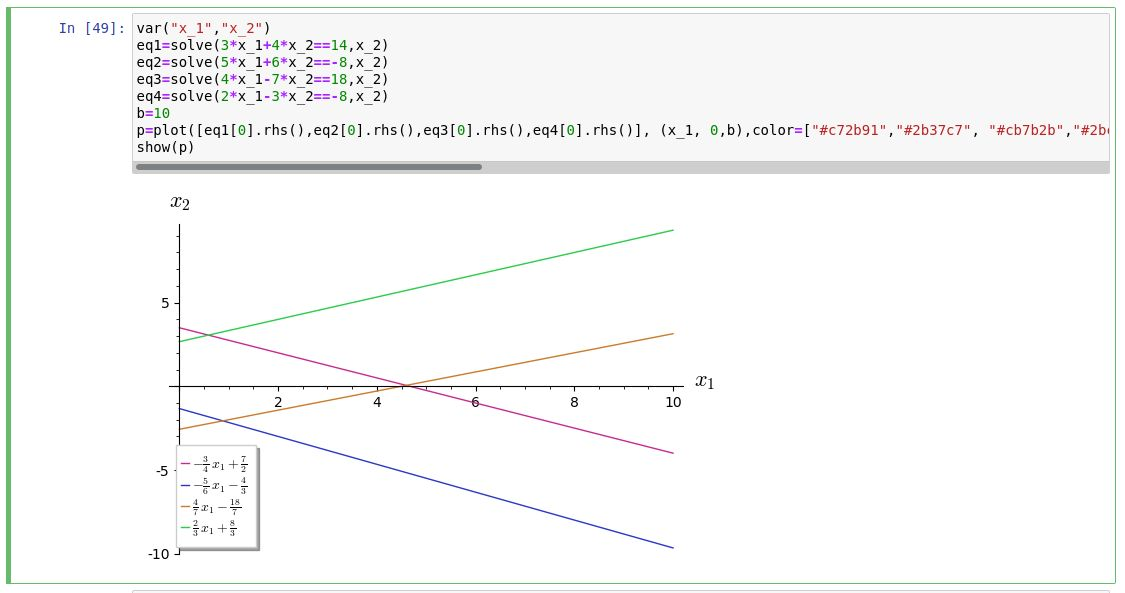# Revision history [back]

I fiddled around and got it to work by changing the legend_label:

var("x_1","x_2")
eq1=solve(3*x_1+4*x_2==14,x_2)
eq2=solve(5*x_1+6*x_2==-8,x_2)
eq3=solve(4*x_1-7*x_2==18,x_2)
eq4=solve(2*x_1-3*x_2==-8,x_2)
b=10
p=plot([eq1.rhs(),eq2.rhs(),eq3.rhs(),eq4.rhs()], (x_1, 0,b),color=["#c72b91","#2b37c7", "#cb7b2b","#2bcb4b"],axes_labels=["$x_1$","$x_2$"],legend_label=[r"$%s$"%latex(eq1.rhs()),r"$%s$"%latex(eq2.rhs()),r"$%s$"%latex(eq3.rhs()),r"$%s$"%latex(eq4.rhs())])
show(p)


This is the result:Since there are several equations in the legend, I use a list. Looking at that part of the code, r"$%s$"%latex(eq1.rhs()) accomplishes the task by first getting the latex code for eq1.rhs() with latex(eq1.rhs()) followed by inserting that in for the string r"$%s$. The r is for a raw string and since its a formula, LaTeX needs it between dollar signs.

I fiddled around and got it to work by changing the legend_label:

var("x_1","x_2")
eq1=solve(3*x_1+4*x_2==14,x_2)
eq2=solve(5*x_1+6*x_2==-8,x_2)
eq3=solve(4*x_1-7*x_2==18,x_2)
eq4=solve(2*x_1-3*x_2==-8,x_2)
b=10
p=plot([eq1.rhs(),eq2.rhs(),eq3.rhs(),eq4.rhs()], (x_1, 0,b),color=["#c72b91","#2b37c7", "#cb7b2b","#2bcb4b"],axes_labels=["$x_1$","$x_2$"],legend_label=[r"$%s$"%latex(eq1.rhs()),r"$%s$"%latex(eq2.rhs()),r"$%s$"%latex(eq3.rhs()),r"$%s$"%latex(eq4.rhs())])
show(p)


This is the result:Since there are several equations in the legend, I use a list. Looking at that part of the code, r"$%s$"%latex(eq1.rhs()) accomplishes the task by first getting the latex code for eq1.rhs() with latex(eq1.rhs()) followed by inserting that in for the string r"$%s$. The r is for a raw string and since its a formula, LaTeX needs it between dollar signs.

It seems a bit awkward, maybe others have a more efficient way.